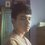# Am I Missing Something?

This is supposed to be one of the easiest problems from BdMO 2014. But I can't seem to find a rigorous way of solving this problem. Am I missing something?

$x$, $a$, $b$ are positive numbers such that if $a>b$, $f(a)>f(b)$ and $f(f(x))=x^2+2$. What is the value of $f(3)$?

This also happens to be my first note!

Thanks!Note by Siam Habib
7 years, 4 months ago

This discussion board is a place to discuss our Daily Challenges and the math and science related to those challenges. Explanations are more than just a solution — they should explain the steps and thinking strategies that you used to obtain the solution. Comments should further the discussion of math and science.

When posting on Brilliant:

• Use the emojis to react to an explanation, whether you're congratulating a job well done , or just really confused .
• Ask specific questions about the challenge or the steps in somebody's explanation. Well-posed questions can add a lot to the discussion, but posting "I don't understand!" doesn't help anyone.
• Try to contribute something new to the discussion, whether it is an extension, generalization or other idea related to the challenge.
• Stay on topic — we're all here to learn more about math and science, not to hear about your favorite get-rich-quick scheme or current world events.

MarkdownAppears as
*italics* or _italics_ italics
**bold** or __bold__ bold
- bulleted- list
• bulleted
• list
1. numbered2. list
1. numbered
2. list
Note: you must add a full line of space before and after lists for them to show up correctly
paragraph 1paragraph 2

paragraph 1

paragraph 2

[example link](https://brilliant.org)example link
> This is a quote
This is a quote
    # I indented these lines
# 4 spaces, and now they show
# up as a code block.

print "hello world"
# I indented these lines
# 4 spaces, and now they show
# up as a code block.

print "hello world"
MathAppears as
Remember to wrap math in $$ ... $$ or $ ... $ to ensure proper formatting.
2 \times 3 $2 \times 3$
2^{34} $2^{34}$
a_{i-1} $a_{i-1}$
\frac{2}{3} $\frac{2}{3}$
\sqrt{2} $\sqrt{2}$
\sum_{i=1}^3 $\sum_{i=1}^3$
\sin \theta $\sin \theta$
\boxed{123} $\boxed{123}$

## Comments

Sort by:

Top Newest

$f(f(1))=3$ => $f(1)=$ $1$ or $2$ or $3$. Easy investigation gives

$f(1) =2$.

$f(f(1))= f(2)= 3$.

$f(f(2))=f(3)= 2^2 +2 =6$.

- 7 years, 4 months ago

Log in to reply

I think you ought to prove $f(n)>n$ for this

- 7 years, 4 months ago

Log in to reply

If $f(1)= 1$ then $f(f(1))= f(1) = 1$. But $f(f(1))=3$ If $f(1)=3$ then $f(f(1))=f(3) = 3$ So, $f(f(3)) = f(3)= 3$ But $f(f(3))=11$

So, $f(1)=2$.

- 7 years, 4 months ago

Log in to reply

I don't understand with your solving.. f(1)=f(2)=1/2/3=3/2=3.. am i wrong to understand??

- 7 years, 4 months ago

Log in to reply

Sorry. I was saying $f(1)=$ 1 or 2 or 3.

- 7 years, 4 months ago

Log in to reply

And how you conclude that f(1)=1or2or3?? and than continue to f(1)=2? sorry i just learned this..

- 7 years, 4 months ago

Log in to reply

Congrats on your first note Siam! It was a good one.

Staff - 7 years, 4 months ago

Log in to reply

×

Problem Loading...

Note Loading...

Set Loading...